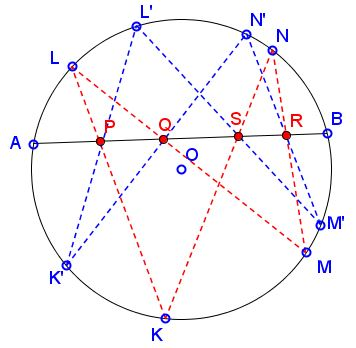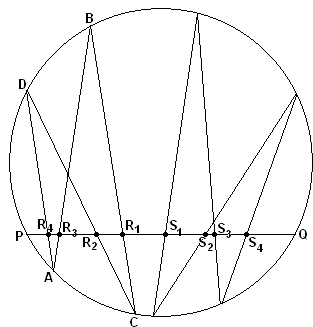# Two Butterflies TheoremWhat is it about? A Mathematical Droodle

Explanation### Two Butterflies Theorem

The theorem below that might be called Two Butterflies Theorem is a generalization of a better known Butterfly Theorem.Let two self-intersecting quadrilaterals $KLMN$ and $K'L'M'N'$ be inscribed into the same circle. Assume the two intersect chord $AB$ at points $P,$ $Q,$ $R,$ $S$ and $P',$ $Q',$ $R',$ $S',$ respectively. $(P$ lies on $KL,$ $Q$ on $LM,$ etc.) Assume also that none of the vertices of the quadrilaterals coincides with either $A$ or $B.$ If some three of the points $P,$ $Q,$ $R,$ $S$ coincide with three corresponding points out of $P',$ $Q',$ $R',$ $S'$ (e.g., $P = P',$ $Q = Q',$ $R = R'),$ then the remaining two points also coincide.

### Proof

As the main instrument in the proof, we' ll use a lemma that served as a basis for one of the proofs of the Butterfly theorem. According to that lemma, the circle at hand is defined by the equation $f = 0,$ where

$f = \lambda l_{KL}l_{MN} + \mu l_{KN}l_{ML},$ and also
$f = \lambda' l_{K'L'}l_{M'N'} + \mu ' l_{K'N'}l_{M'L'}.$

Consider the line $AB$ as a number line with a coordinate $x.$ Assume for definiteness sake that $P = P',$ $Q = Q',$ and $R = R'.$ Assume also that the points $P,$ $Q,$ $R,$ $S,$ and $S'$ have coordinates $p,$ $q,$ $r,$ $s,$ and $s',$ respectively. Then, restricted to the line $AB,$ the above equations imply

$\alpha (x - p)(x - r) + \beta (x - q)(x - s) = \alpha '(x - p)(x - r) + \beta '(x - q)(x - s').$

We want to show that $s = s'.$

If $\alpha = \alpha ',$ then also $\beta = \beta '$ and $s = s'$ right away. But, by our convention $(P$ lies on $KL,$ $Q$ on $LM,$ etc.), $Q$ may not equal either $P$ or $R,$ therefore, $(x - p)(x - r)$ is not divisible by $(x - q).$ Therefore, necessarily $\alpha = \alpha ',$ and we are finished.There is an additional proof due to Nathan Bowler.

Let $P$ be a point not on a circle $C.$ Let $f_{P}$ be the transformation taking each point $A$ on $C$ to the other intersection of $AP$ with $C.$ Then there is a mobius transform $g_{P}$ such that $f_{P}$ is the restriction of $g_{P}$ to $C.$

### Proof

If $P$ lies outside $C,$ then by the intersecting chords theorem we may take $g_{P}$ to be inversion in a circle of suitable radius centred at $P.$ If $P$ lies inside $C,$ it is merely necessary to add an extra half-twist centred at $P.$

Now let $g$ be the mobius transformation obtained by composing $g_{P},$ $g_{Q},$ $g_{R}$ and $g_{S}.$ Then $g$ has three fixed points on $C:$ $A,$ $B$ and $K.$ So $g$ is the identity on $C$ and so $g(K') = K'.$ The result follows.

Note that the same argument will give the following interesting porism as a generalisation:

Let $A$ and $B$ lie on a circle $S,$ and let $P_{1}, \ldots, P_{2n}$ be points on $AB$ distinct from $A$ and $B.$ If there is any point $K$ on $S,$ other than $A$ or $B,$ with the property that there are points $K_{1}, \ldots, K_{2n+1}$ on $S$ with $P_{i}$ lying on $K_{i} K_{i+1}$ for all $i\le 2n,$ and $K_{1} = K_{2n+1} = K,$ then all points on $S$ have this property.

### Remark

We may generalise still further. The key point to note is that in the lemma we may in fact construct $g_{P}$ knowing only $P,$ $A$ and $B$ where $P,$ $A$ and $B$ are collinear and $A$ and $B$ lie on the circle $C.$ Then, clarifying the proof by noting that $g$ preserves the orientation of the plane and so is not the identity merely on $C$ but everywhere, we may observe that it is not necessary for the circles $KLMN$ and $K'L'M'N'$ to be the same; we need only require that both pass through both $A$ and $B.$

To be less formal, in the two butterflies theorem the two butterflies may live on different circles, provided that those circles meet at $A$ and $B.$

As a further generalization, it comes out that the two circles need not intersect: the theorem remains valid as long as $A$ and $B$ lie on the radical axis of the two circles.

Finally, like, say, Pascal's theorem, the theorem discussed here, deals only with incidence of points and lines and, therefore, is true for any non-degenerate conic. We have a demonstration of that statement for ellipses along with a demonstration of porism suggested by Nathan Bowler.We have an additional proof of the main result. The proof is by L. Hoehn of a statement first proved by D. Jones.With a reference to the above diagram, Jones' result appears as

Let $PQ$ be a fixed chord of a circle and let "butterfly $R$" and "butterfly $S$" be inscribed in the circle and oriented such that their wings cut $PQ$ (in order from left to right) at $R_{4},$ $R_{3},$ $R_{2},$ $R_{1}$ and $S_{1},$ $S_{2},$ $S_{3},$ $S_{4},$ respectively. If $PR_{1} = QS_{1},$ $PR_{2} = QS_{2}$ and $PR_{3} = QS_{3},$ then $PR_{4} = QS_{4}.$

(A reflection in the perpendicular bisector of $PQ$ immediately shows that the "Double Butterfly Theorem" is equivalent to the "Two Butterflies Theorem".)

We apply Hiroshi Haruki's Lemma twice.

For the proof of the theorem we let $m = PR_{4},$ $n = QS_{4},$ $a = PR_{3} = QS_{3},$ $b = R_{3}R_{2} = S_{3}S_{2},$ $c = R_{2}R_{1} = S_{2}S_{1},$ $d = R_{1}S_{1},$ and $e = a + b + c + d.$

By applying Haruki's lemma twice to points $A$ and $B$ and fixed chords $PQ$ and $CD$ of butterfly $R,$ we have

$m\cdot (b + c + e) / (a - m) = (a + b)\cdot e / c,$

sine both are equal to the same constant. Similarly,

$n\cdot (b + c + e) / (a - n) = (a + b)\cdot e / c,$

for butterfly $S.$ By transitivity of equality, we easily get $m = n,$ which completes the proof.

### References

1. D. Jones, A Double Butterfly Theorem, Math Magazine 49 (1976), pp. 86-87
2. L. Hoehn, A New Proof of the Double Butterfly Theorem, Math Magazine 63 (1990), pp. 256-257
3. V. V. Prasolov, Essays On Numbers And Figures, AMS, 2000### Butterfly Theorem and Variants